# Pontryagin space

(diff) ← Older revision | Latest revision (diff) | Newer revision → (diff)

A Hilbert space with an indefinite metric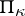that has a finite rank of indefiniteness. Basic facts concerning the geometry of these spaces were established by L.S. Pontryagin . Besides the facts common for spaces with an indefinite metric, the following properties hold.

If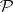is an arbitrary non-negative linear manifold in, then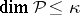; ifis a positive linear manifold and, then its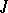-orthogonal complementis a negative linear manifold and. Moreover,is a complete space with respect to the norm. If the linear manifold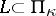is non-degenerate, then its-orthogonal complementis non-degenerate as well and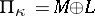.

The spectrum (in particular, the discrete spectrum) of a-unitary (-self-adjoint) operator is symmetric with respect to the unit circle (real line), all elementary divisors corresponding to eigen values,, are of finite order,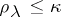,. The sum of the dimensions of the root subspaces of a-unitary (-self-adjoint) operator corresponding to eigen values,(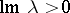), does not exceed.

The following theorem  is fundamental in the theory of-self-adjoint operators on a Pontryagin space: For each-self-adjoint operator(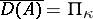) there exists a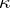-dimensional (maximal) non-negative invariant subspace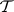in which all eigen values ofhave non-negative imaginary parts, and a-dimensional non-negative invariant subspace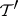in which all eigen values have non-positive imaginary parts. A similar statement in which the upper (lower) half-plane is replaced by the exterior (interior) of the unit disc is also valid for-unitary operators, and under certain additional conditions — even for operators on the space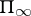.

Ifis a-unitary operator, then its maximal invariant subspaces,can be chosen so that the elementary divisors of the operator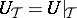,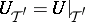are of minimal order. In order that a polynomialwith no roots inside the unit disc has the property:,, it is necessary and sufficient that it can be divided by the minimal annihilating polynomial of the operator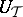. Ifis a cyclic operator, then its non-negative invariant subspaces of dimensionare uniquely determined. In this case the above-mentioned property of the polynomialwith roots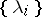outside the unit disc,, is equivalent to the divisibility ofby the characteristic polynomial of.

Each completely-continuous-self-adjoint operatoron a Pontryagin spacesuch that zero belongs to its continuous spectrum does not have a residual spectrum. The root vectors of such an operator form a Riesz basis inwith respect to the (definite) norm.

Many facts concerning invariant subspaces and the spectrum can be generalized to a case of-isometric and-non-expanding operators. Thus, if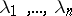is an arbitrary set of eigen values of a-isometric operator,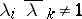,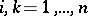, and if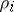is the order of the elementary divisor at the point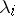, then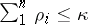. Any-non-expanding boundedly-invertible operatorhas a-dimensional invariant non-negative subspacesuch that all eigen values of the restriction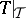lie in the unit disc . A similar fact holds for maximal-dissipative operators. In general, a-dissipative operator,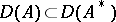, has at mosteigen values in the upper half-plane.-isometric and-symmetric (and more generally,-non-expanding and-dissipative) operators are related by the Cayley transformation (cf. Cayley transform), which has onall natural properties . This fact allows one to develop the extension theory simultaneously for-isometric and-symmetric operators. In particular, every-isometric (-symmetric) operator can be extended to a maximal one. If its deficiency indices are different, then it has no-unitary (-self-adjoint) extensions. If these indices are equal and finite, then any maximal extension is-unitary (-self-adjoint).

For completely-continuous operators on, a number of statements on the completeness of the system of root vectors, analogous to the corresponding facts from the theory of dissipative operators on spaces with a definite metric, is valid.

How to Cite This Entry:
Pontryagin space. Encyclopedia of Mathematics. URL: http://encyclopediaofmath.org/index.php?title=Pontryagin_space&oldid=11492
This article was adapted from an original article by N.K. Nikol'skiiB.S. Pavlov (originator), which appeared in Encyclopedia of Mathematics - ISBN 1402006098. See original article